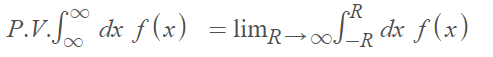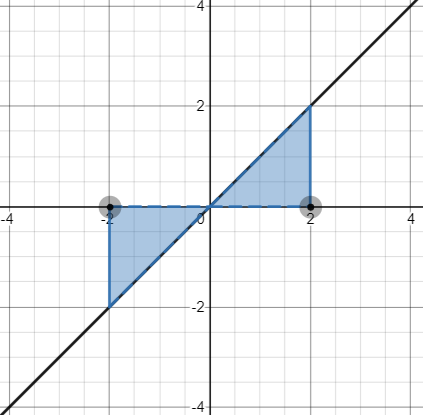# Cauchy Principal Value: Definition

## What is Cauchy Principal Value?

Cauchy’s Principal Value Method assigns values to a divergent integral by making the assumption that each limit converges at the same rate. Roughly speaking, you symmetrically whittle down integration on a singularity or at infinity. In notation, Cauchy’s Principal Value (P.V.) is written as:The difference between the Riemann improper integral and the Cauchy principal value is that the limits with the P.V. tend to infinity at the same rate. With the Riemann improper integral, there are two independent limits (A → -∞ and B → ∞).

## When Does the Cauchy Principal Value Work?

Cauchy Principal Value works even if the interval isn’t symmetric or if you don’t have an odd integrand. The method only works if applying it to a convergent integral doesn’t change the value of that integral ; Convergence of an improper integral implies that a Cauchy P.V. exists. However, existence of a P.V. does not imply that the integral converges. In fact, it’s possible to have completely different solutions (e.g. convergence to zero for the P.V. compared to convergence to infinity for the actual integral).

As an example, consider the odd function f(x) = x.The integral of the function f(x) = x is zero between two points -a and a.

The doubly-improper integraldoes not converge. However, the Cauchy Principal Value does exist . For any value of a,## References

Graph: Desmos.com
 Wheeler, J. Assigning Value to the Valueless… The Cauchy Principal Value Method and Related Ideas in Divergent Series. Retrieved July 8, 2021 from: http://www.pitt.edu/~jwheeler/Principal%20Values.pdf
 Beerends, R. & Morsche, H. (2003). Fourier and Laplace Transforms. Cambridge University Press.

CITE THIS AS:
Stephanie Glen. "Cauchy Principal Value: Definition" From StatisticsHowTo.com: Elementary Statistics for the rest of us! https://www.statisticshowto.com/cauchy-principal-value/